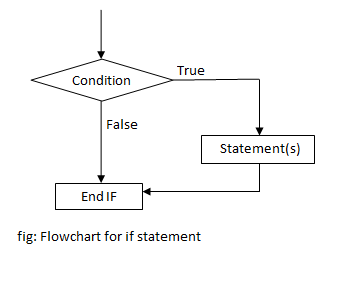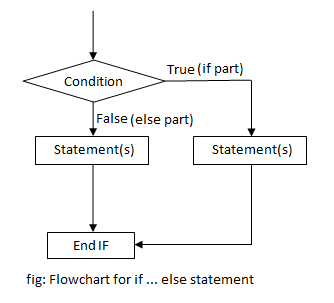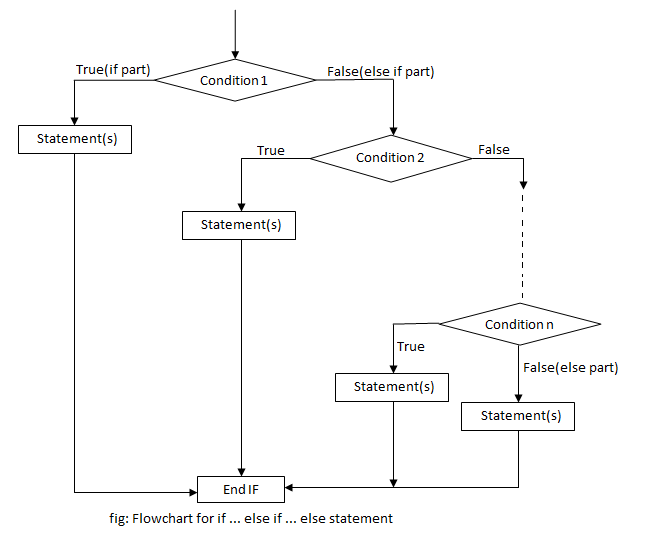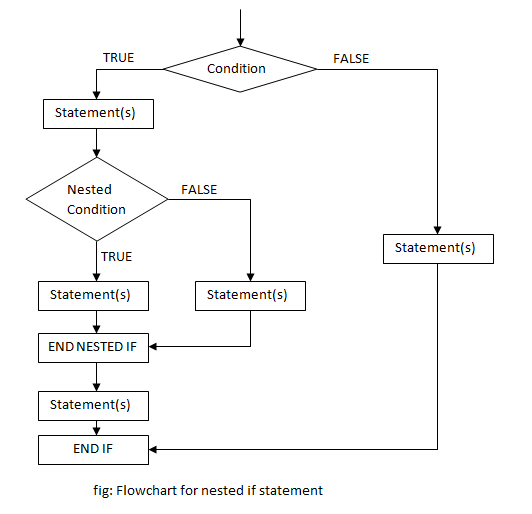# if statements in C++ Programming

While writing computer programs, we need to check certain condition to instruct the program to branch accordingly. Like most of the programming languages, C++ has if statement to check the condition and make decision. Based on the number of conditions to be checked, we have different types of if statement. They are

## if statement

if statement is a conditional statement which is used to make decision. It is used when a single condition is to be checked. A condition is enclosed in if statement which decides the sequence of execution of instruction. If the condition is true, the statements inside if statement are executed, otherwise they are skipped.

### Syntax of if statement

```if (condition)
{
statements;
... ... ...
}```

### Flowchart for if statement### Example of if statement

C++ program to check percentage of a student and display pass if it is greater than 40.

```#include <iostream>
#include <conio.h>
using namespace std;

int main()
{
float percent;
cin>>percent;
cout<<"You scored "<<percent<<"%"<<endl;
if (percent>=40)
{
cout<<"Congratulation: You have passed";
}
getch();
return 0;
}```

In this program, the percentage of a student is entered by user and a message saying how much the student scored is displayed. If the student has scored more than or equal to 40%, he/ she is passed and a congratulation message is displayed on the output screen.

Output

```Enter your percentage: 71
You scored 71%
Congratulation: You have passed```
```Enter your percentage: 34
You scored 34%
```

## if … else statement

if … else statement is a two way branching statement. It is similar to if statement but the only difference is if the condition is false then a different block of statements are executed which is inside else statement.

### Syntax of if…else statement

```if (condition)
{
statements;
... ... ...
}
else
{
statements;
... ... ...
}
```

### Flowchart for if … else statement### Example of if … else statement

C++ program to check percentage of a student and display pass or fail.

```#include <iostream>
#include <conio.h>
using namespace std;

int main()
{
float percent;
cin>>percent;
cout<<"You scored "<<percent<<"%"<<endl;
if (percent>=40)
{
cout<<"Congratulation: You have passed";
}
else
{
cout<<"Sorry: You have failed";
}
getch();
return 0;
}```

This program asks the percentage of student. If the percentage is equal to or greater than 40 then a congratulation message saying you have passed is displayed. Otherwise, i.e if percentage is below 40 then a sorry message is printed on the output screen.

Output

```Enter your percentage: 86
You scored 86%
Congratulation: You have passed```
```Enter your percentage: 37
You scored 37%
Sorry: You have failed```

## if … else if … else statement

if … else if … else statement is used for multiple branching. When there are two or more conditions that needs to be checked to decide which block of statement is to be executed, it is used. The number of else if statements depends upon the number of conditions to be checked.

### Syntax of if…else if…else statement

```if (condition 1)
{
statements;
... ... ...
}
else if (condition 2)
{
statements;
... ... ...
}
... ... ...
... ... ...
else if (condition n)
{
statements;
... ... ...
}
else
{
statements;
... ... ...
}
```

### Flowchart for if … else if … else statement### Example of if … else if … else statement

C++ program to check percentage of a student and display division(distinction, first, second, third or fail).

```#include <iostream>
#include <conio.h>
using namespace std;

int main()
{
float percent;
cin>>percent;
cout<<"You scored "<<percent<<"%"<<endl;
if (percent>=80)
{
cout<<"You have passed with distinction";
}
else if (percent>=60)
{
cout<<"You have passed with first division";
}
else if (percent>=50)
{
cout<<"You have passed with second division";
}
else if (percent>=40)
{
cout<<"You have passed with third division";
}
else
{
cout<<"Sorry: You have failed";
}
getch();
return 0;
}```

This program asks the percentage of a student and display which division he/she has got. The criteria for division is displayed below:

 Percentage Division >=80 Distinction >=60 and <80 First division >=50 and <60 Second Division >=40 and <50 Third Division <40 Fail

According to the condition, the result is displayed.

Output

```Enter your percentage: 87.67
You scored 87.67%
You have passed with distinction```
```Enter your percentage: 34.50
You scored 34.5%
Sorry: You have failed```
```Enter your percentage: 45.83
You scored 45.83%
You have passed with third division```

## Nested if statements

An if statement inside another if statement is known as nested if statements. Nested if statements are used if there is a sub condition to be tested after one condition has been checked. The depth/number of nested if statements depends upon the number of conditions to be checked.

### Syntax of nested if statement

```if (condition 1)
{
statements;
if (sub condition 1)
{
statements;
}
statements;
}
else if (condition 2)
{
statements;
if (sub condition 2)
{
statements;
}
statements;
}
... ... ...
... ... ...
else
{
statements;
if (sub condition n)
{
statements;
}
statements;
}```

### Flowchart for nested if statement### Example of nested if statement

C++ program to check if a number entered by user is even and divisible by 5 or not.

```#include <iostream>
#include <conio.h>
using namespace std;

int main()
{
int n;
cout<<"Enter a number: ";
cin>>n;
if (n%2 == 0)
{
if (n%5 == 0)
{
cout<<"Number is even and divisible by 5";
}
else
{
cout<<"Number is even but not divisible by 5";
}
}
else
{
if (n%5 == 0)
{
cout<<"Number is not even but divisible by 5";
}
else
{
cout<<"Number is not even and not divisible by 5";
}
}
}```

This program checks whether a number is even and divisible by 5 or not. A number is entered by user which is then checked by using nested if statements.

Output

```Enter a number: 6
Number is even but not divisible by 5```
```Enter a number: 20
Number is even and divisible by 5
```